﻿ 基于ARIMA模型的宿州市GDP分析与预测 Analysis and Forecast of Suzhou’s GDP Based on ARIMA Model

Statistics and Application
Vol.07 No.03(2018), Article ID:25598,10 pages
10.12677/SA.2018.73040

Analysis and Forecast of Suzhou’s GDP Based on ARIMA Model

Pei Wang

China University of Mining and Technology, Xuzhou Jiangsu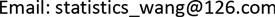Received: Jun. 3rd, 2018; accepted: Jun. 20th, 2018; published: Jun. 27th, 2018ABSTRACT

Gross Domestic Product (GDP), as an important indicator of national economic operation and monitoring, plays an important role in guiding macroeconomic policies for party committees and governments. This paper selects Suzhou’s GDP data from 1978 to 2017, using time series analysis methods and R software. The establishment of an ARIMA model of Suzhou’s GDP and a forecast of the Suzhou’s GDP from 2018 to 2020 provide a reference for the Suzhou Municipal Party Committee and municipal government to formulate economic development goals and macroeconomic decision-making.

Keywords:GDP, ARIMA Model, ForecastCopyright © 2018 by author and Hans Publishers Inc.1. 引言

2. ARIMA模型的结构

ARIMA模型本质上就是差分运算与ARMA模型的组合。考虑非平稳序列 $\left\{{x}_{t}\right\}$ ，如果该非平稳序列能通过d次差分后变为平稳序列，则满足如下结构的模型称为ARIMA(p,d,q)模型：

$\left\{\begin{array}{c}\Phi \left(B\right){\nabla }^{d}{x}_{t}=\Theta \left(B\right){\epsilon }_{t}\\ E\left({\epsilon }_{t}\right)=0,Var\left({\epsilon }_{t}\right)={\sigma }_{\epsilon }^{2},E\left({\epsilon }_{t}{\epsilon }_{s}\right)=0,s\ne t\\ E\left({\epsilon }_{t}{\epsilon }_{s}\right)=0,\forall s

3. ARIMA模型的建立与检验

3.1. ARIMA模型建立的方法

3.2. 数据的来源与描述

3.3. 序列的平稳化处理

3.4. 模型建立与检验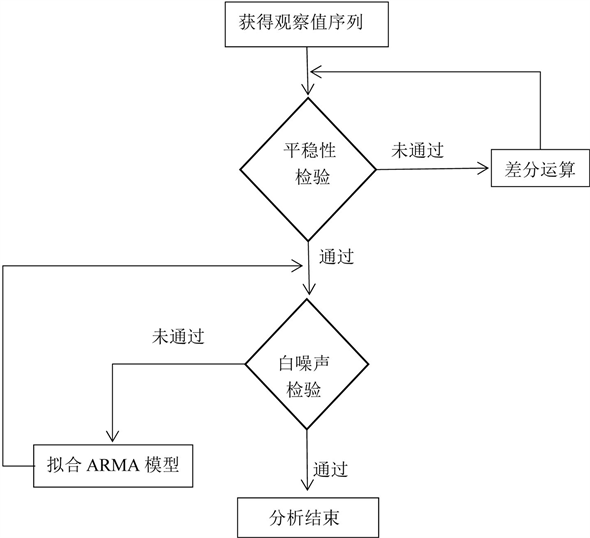Figure 1. Modeling processTable 1. Statistical table of Suzhou's gross domestic product (GDP) from 1978 to 2017 (100 million yuan)Figure 2. Suzhou’s gross domestic product (GDP) time series from 1978 to 2017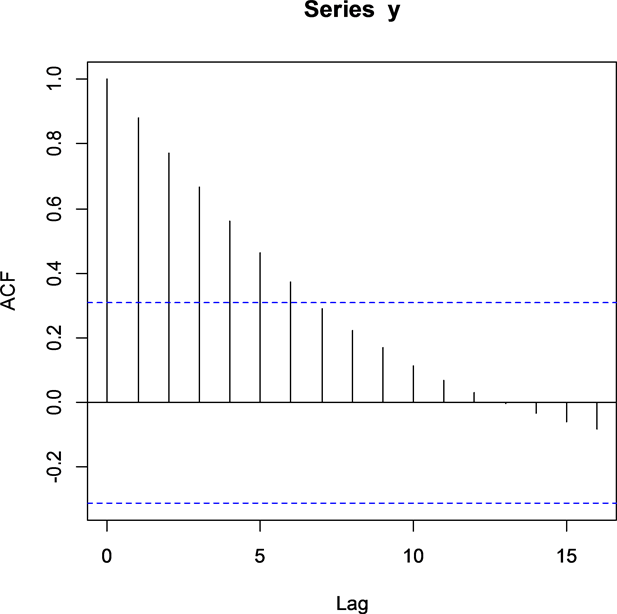Figure 3. Time series ACF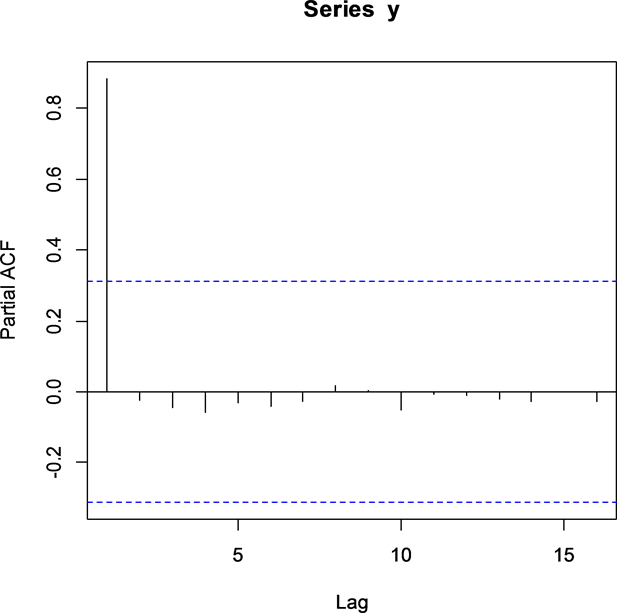Figure 4. Time series PACFFigure 5. Time series first-order differentialFigure 6. Time series second-order differentialTable 2. ADF Single root test results of Suzhou’s GDP first-order differential sequencesTable 3. ADF single root test results of Suzhou’s GDP second-order differential sequences

LB统计量p-Value均显著大于0.05，可以知道残差序列通过了纯随机性检验，即认为以上模型显著有效  。

3.5. 模型优化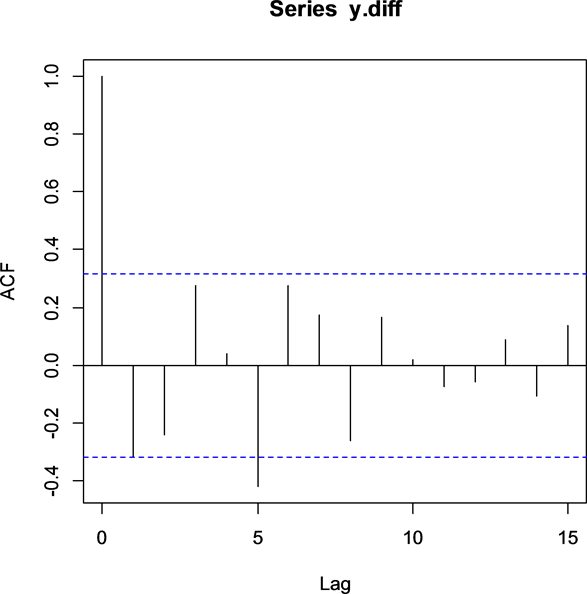Figure 7. Second-order differential ACF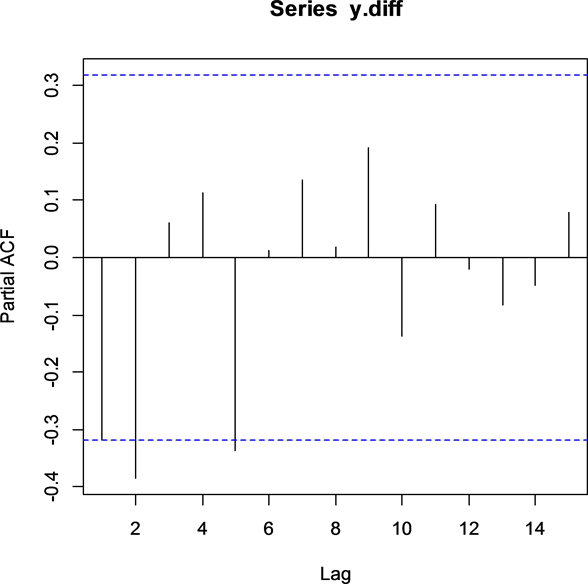Figure 8. Second-order differential PACF

4. 模型的预测Table 4. Suzhou’s GDP sequence model significance test resultsTable 5. Using ARIMA (2,2,2) to predict the GDP results of Suzhou from 2018 to 2020Figure 9. Second-order differential PACF

5. 结论

1) 从图9可以看出，未来三年宿州市GDP会保持平稳快速增长，增速略有下降，这与我国经济发展从高速发展转向高质量发展确实基本吻合，宿州市GDP保持中高速增长水平于现阶段宿州市为欠发达地区的发展实际相一致。

2) 通过对宿州市1978年至2017年GDP序列进行分析和建模，可以说明ARIMA模型在拟合非平稳时间序列时具有很好的效果。本文所建立的ARIMA(2,2,2)，可用于宿州市GDP的短期预测，为宿州市制定经济发展目标提供参考。

Analysis and Forecast of Suzhou’s GDP Based on ARIMA Model[J]. 统计学与应用, 2018, 07(03): 340-349. https://doi.org/10.12677/SA.2018.73040

1. 1. 王燕. 时间序列分析: 基于R [M]. 北京: 中国人民大学出版社, 2015.

2. 2. 龚国勇. ARIMA模型在深圳GDP预测中的应用[J]. 数学的实践与认识, 2008, 38(4): 53-57.

3. 3. Wei, N., Bian, K.J. and Yuan, Z.F. (2010) Analysis and Forecast of Shaanxi GDP Based on ARIMA Model. Asian Agricultural Research, 2, 34-36, 41.

4. 4. 郎星宇, 李鑫, 孙弋茗, 白晓东. 基于ARIMA模型对中国1953~2015年GDP的分析建模及预测[J]. 统计学与应用, 2017, 6(2): 219-230.

5. 5. Wabomba, M.S., Mutwiri, M.P. and Fredrick, M. (2016) Modeling and Forecasting Kenyan GDP Using Autoregressive Integrated Moving Average (ARIMA) Modeling. Science Journal of Applied Mathematics and Statistics, 4, 64-73. https://doi.org/10.11648/j.sjams.20160402.18

6. 6. 蒋燕. ARIMA模型在广西全社会固定资产投资预测中的应用[J]. 数理统计与管理, 2006, 25(5): 588-592.

7. 7. 郭晓峰. 基于ARIMA模型的中国CPI走势预测分析[J]. 统计与决策, 2012(11): 29-32.

8. 8. 池启水. 中国石油消费量增长趋势分析——基于ARIMA模型的预测与分析[J]. 资源科学, 2007, 29(5): 69-73.

9. 9. 李娜, 薛俊强. 基于最优ARIMA模型的我国GDP增长预测[J]. 统计与决策, 2013(9): 23-26.

R语言编程程序

y<-ts(x$gdp,start=1978) plot(y) acf(y) pacf(y) y.dif<-diff(y) plot(y.dif) library(fBasics) library(fUnitRoots) for(i in 1:3)print(adfTest(y.dif,lag=i,type=c)) y.diff<-diff(y.dif) plot(y.diff) for(i in 1:3)print(adfTest(y.diff,lag=i,type=c)) acf(y.diff) pacf(y.diff) library(zoo) library(forecast) auto.arima(y) y.fit1<-arima(y,order=c(1,2,1)) y.fit1 for(i in 1:2)print(Box.test(y.fit1$residual),lag=6*i)

y.fit2<-arima(y,order=c(2,2,1))

y.fit2

for(i in 1:2)print(Box.test(y.fit2$residual),lag=6*i) y.fit3<-arima(y,order=c(0,2,1)) y.fit3 for(i in 1:2)print(Box.test(y.fit3$residual),lag=6*i)

y.fit4<-arima(y,order=c(1,2,2))

y.fit4

for(i in 1:2)print(Box.test(y.fit4$residual),lag=6*i) y.fit5<-arima(y,order=c(2,2,2)) y.fit5 for(i in 1:2)print(Box.test(y.fit5$residual),lag=6*i)

y.fit6<-arima(y,order=c(0,2,2))

y.fit6

for(i in 1:2)print(Box.test(y.fit6\$residual),lag=6*i)

y.fore<-forecast(y.fit5,h=3)

y.fore